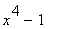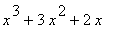CH033 - Mathematics for Chemists

Elementary Algebra (i) (1) Topics coverec in this sheet
(2) Recall
(3) Exercises

Note: The solutions for the qestion no. 3b may be dowloaded from here.

[ TOP ]
(1) Topics covered in this sheet:
• Introduction to the f(x) notation
• Factorisation
• Difference of two squares
• Difference and sum of of two cubes
• Factor theorem
• Soving simple equations
• The concept of real/non-real roots

[ TOP ]
(2) Recall:

(a) Nomenclature

(i) Symbols(ii) Sets(iii) Numbers(iv) Note that:(b) Sums/Differences of two squares/cubes.(c) Definition:
We say that  f(x) is a polynomial in x of order n whenand.
(d) Factor Theorem:
• If (x-a) is a factor of f(x) then f(a) = 0.
• Conversly, for a given f(x) = a polynomial in x, if f(a) = 0 then (x-a) is a factor of f(x).
(e) Real/non-real factors/roots
Consider f(x)nth order polynomial in x with rael cofficients ci given by:• f(x) will have a maxium of n real roots and n real factors.
• f(x) may also have some complex roots. If this is the case, these complex roots must occur in pairs of complex conjugates. The total number of real/complex roots is always equal to n (taking into considerations any double roots!). Thus for example:
• A cubic may have: (i) 3 real roots, or (ii) 1 real root and two complex roots which have to be complex conjgates.
• A quardic may have: (i) four real roots, (ii)  2 real roots and 2 complex roots which have to be complex conjgates, or (iii) 4 complex roots, i.e. two pairs of complex conjgates.
• When n is ODD, we are guanateed atb least one real root. This is not the case when n is EVEN.

[ TOP ]
(3) Qustions:

(a) Evaluate (i)  f (2) , (ii)  f (-1), (iii) f (-3)  and (iv)  f (0);
(b) Factorise f(x) as completly as possible.
(c) Solve the equations f(x) = 0
... given that f(x) is given by:

[ TOP ]

 No. f (x) 123456789101112131415161718192021222324252627282930313233343536373839404142434445464748495051525354555657585960[ TOP ]